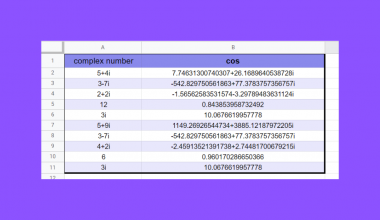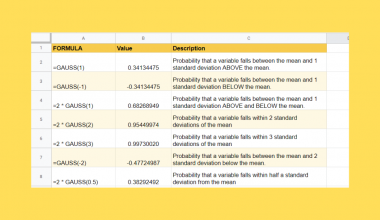# How to Use FORMULATEXT Function in Google Sheets

The `FORMULATEXT` function in Google Sheets is useful when you need to extract formulas from a cell as a text string.

Cells passed through this function output the formula rather than the final value of the cell.

The rules for using `FORMULATEXT` in Google Sheets are as follows:

• The function takes a cell as an argument then returns what is displayed in the formula bar when selecting that cell
• If the cell simply contains text, then the function will return an error.

Let’s look at a quick example.

As I was finalizing my monthly expense tracker on Google Sheets, I’ve noticed that my various expenses are all computed differently.

Some expenses, like my food and transportation totals, required me to import data from another sheet. Other expenses required some division since I would be splitting the cost with a roommate of mine. And some expenses, like my Internet bill, I simply need to input into the tracker manually.

With all these different ways of computing expenses, it becomes a bit difficult to see right away where all these totals were coming from.

With the `FORMULATEXT` function, I was able to see at a glance how each of my totals was computed. I can see which expenses get data from another sheet and which expenses are derived from other cells in my worksheet. Now it is much easier to get a sense of how I arrive at my totals.

My use case is just one way to use the `FORMULATEXT` function in Google Sheets.  We can also use the function as an easy way to check which cells in a worksheet are constants. Another possible use case is to highlight a specific formula used in a cell, especially when a computation is not quite straightforward.

With those uses in mind, it’s clear that the `FORMULATEXT` function is a great way to reveal the computations that are happening on your worksheets.

Let’s learn how to write the `FORMULATEXT` function ourselves in Google Sheets and later use actual values and formulas to see this function in action.

## The Anatomy of the FORMULATEXT Function

So the syntax (the way we write) the `FORMULATEXT` function is as follows:

`=FORMULATEXT(cell)`

Let’s dissect this thing and understand what each of these terms means:

• =‘ the equal sign is just how we start any function in Google Sheets.
• FORMULATEXT()‘ is our `FORMULATEXT` function. `FORMULATEXT` will output the formula found within that cell or cell range. `FORMULATEXT()` will only evaluate the top left most cell in that range if a cell range is provided. However, if the provided cell references itself, `FORMULATEXT()` will handle that circular reference and simply output the formula written in that cell. Lastly, if the provided cell is not a valid formula, then `FORMULATEXT()` will return a ‘#N/A’ error.
• cell‘ refers to the cell you want to verify as containing a formula.

## A Real Example of Using FORMULATEXT Function

Take a look at the example below to see how `FORMULATEXT` functions are used in Google Sheets.As you can see the `FORMULATEXT` function reveals the formulas used by each cell in Column B.

The first example outputs a #N/A error since the value was inputted manually and is not a formula. An easy way to know what counts as a formula is to remember that every formula begins with an equal sign. For instance, the next two expenses are both just numbers, but are still considered as a formula since it begins with an equal sign.

Alternatively, you can use the `ISFORMULA` function if you only want to determine whether a cell contains a formula or not. This function however only outputs a TRUE or FALSE value, and not the actual formula like `FORMULATEXT`.

The `FORMULATEXT` function also reveals to us how we were able to compute the Transportation, Electricity, Water, and Food expenses. At a glance we are able to see that the Food total in B8 references another sheet, and that the Transportation total is just a sum of cells B3 and B4.
You may make a copy of the spreadsheet using the link I have attached below.

Since the `FORMULATEXT` function returns text, we can combine this function with other functions to take advantage of the result.

For instance, the following formula can detect whether a particular cell uses the SUM function in their formula.

``=REGEXMATCH(FORMULATEXT(B5),"SUM")``

You can also use FORMULATEXT to handle errors. The following formula returns the value of a given cell if no errors are detected, otherwise it returns the formula.

``=IF(ISERROR(B2),FORMULATEXT(B2),B2)``

Great! Let’s begin writing our own `FORMULATEXT` function in Google Sheets.

## How to Use FORMULATEXT Function in Google Sheets

1. Before we type our function, let’s add a sample formula for us to retrieve later on. In this example, I used the formula “= 25 * 300 / 5 in cell A2, which evaluates to 1500.2. To start using `FORMULATEXT`, we should select any cell to make it the current active cell. For this guide, cell B2 will be where we will input our function’s result.
3. Next, simply type the equal sign ‘=‘ to begin the function, followed by the name of the function we’ll be using which is ‘formulatext‘ (or ‘FORMULATEXT‘, either is valid in Google Sheets).
4. You should find that the auto-suggest box shows you the `FORMULATEXT` function even before fully typing it out. You may press the Tab button on your keyboard to let Google Sheets auto-fill your Formula Bar with “FORMULATEXT(“.
As seen below, a tooltip box appears with info on the selected function, we simply hit the arrow on the top-right hand corner of the box to minimize it.5. Let’s type out the cell we want to get the formula from, in this case our sample formula is in cell A2. We can type that out in the Formula bar, or we could just click the A2 cell to add it to our formula bar in a single click. Afterwards, simply hit Enter on your keyboard to let the function evaluate.6. If you followed my instructions, cell B2 should read out the formula we typed earlier in cell A2. We have successfully extracted the formula from another cell.
7. Let’s try another example, but this time let’s see what happens when a cell’s value is not a formula. In this example, I’ve typed in 2000 manually in A3.
We can drag down the `FORMULATEXT` formula we typed in earlier by placing the cursor at the bottom-right part of the selected cell (it looks like a small blue square) and dragging it down one cell.8. We did it! An error should appear since the cell we are trying to extract from (A3 this time) is not a valid formula.That’s pretty much all you need to know. The `FORMULATEXT` function is quite easy to grasp since it only involves one argument, the cell you’re trying to extract a formula from.

You can now use the `FORMULATEXT` functions in Google Sheets together with the various other Google Sheets formulas available to create more powerful worksheets for your convenience. Make sure to subscribe if you want to get notified of our future posts.Our goal this year is to create lots of rich, bite-sized tutorials for Google Sheets users like you. If you liked this one, you'll love what we are working on! Readers receive ✨ early access ✨ to new content.

D

##### You May Also Like## How To Use IMCOS Function in Google Sheets

The IMCOS function in Google Sheets is useful when you would like to retrieve the cosine of a…## How to Use LAMBDA Function in Google Sheets

This guide will explain how to use LAMBDA function in Google Sheets. When we want to create a…## How to Use GAUSS Function in Google Sheets

The GAUSS function in Google Sheets is useful when you need to calculate the probability that a random…## How to Use WRAPROWS Function in Google Sheets

This guide will explain how to use the WRAPROWS function in Google Sheets to form a new output. …